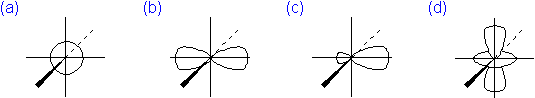# Solution Set 8

$$\newcommand{\vecs}{\overset { \rightharpoonup} {\mathbf{#1}} }$$ $$\newcommand{\vecd}{\overset{-\!-\!\rightharpoonup}{\vphantom{a}\smash {#1}}}$$$$\newcommand{\id}{\mathrm{id}}$$ $$\newcommand{\Span}{\mathrm{span}}$$ $$\newcommand{\kernel}{\mathrm{null}\,}$$ $$\newcommand{\range}{\mathrm{range}\,}$$ $$\newcommand{\RealPart}{\mathrm{Re}}$$ $$\newcommand{\ImaginaryPart}{\mathrm{Im}}$$ $$\newcommand{\Argument}{\mathrm{Arg}}$$ $$\newcommand{\norm}{\| #1 \|}$$ $$\newcommand{\inner}{\langle #1, #2 \rangle}$$ $$\newcommand{\Span}{\mathrm{span}}$$ $$\newcommand{\id}{\mathrm{id}}$$ $$\newcommand{\Span}{\mathrm{span}}$$ $$\newcommand{\kernel}{\mathrm{null}\,}$$ $$\newcommand{\range}{\mathrm{range}\,}$$ $$\newcommand{\RealPart}{\mathrm{Re}}$$ $$\newcommand{\ImaginaryPart}{\mathrm{Im}}$$ $$\newcommand{\Argument}{\mathrm{Arg}}$$ $$\newcommand{\norm}{\| #1 \|}$$ $$\newcommand{\inner}{\langle #1, #2 \rangle}$$ $$\newcommand{\Span}{\mathrm{span}}$$

## Q1

How many valence electrons does an oxygen atom have?

1. 2
2. 6
3. 8
4. 16

A1. B

## Q2

Fluorine atoms tend to...?...when they form chemical compounds with metals.

1. lose electrons
2. gain electrons
3. neither lose nor gain electrons...they usually share electrons equally with metals.
4. Fluorine atoms do not form compounds with other atoms...fluorine is an inert gas.

A2. b

## Q3

The identity of an element is determined by...

1. the number of its protons.
2. the number of its neutrons.
3. the number of its electrons.
4. its atomic mass.

A3. A

## Q4

The probability area that best represents the shape of an atomic 3p orbital is...A4. b

## Q5

Which of the following is the correct electron-dot formula for water?A5. a

## Q6

Which of the following is the correct electron-dot formula for carbon dioxide?A6. c

## Q7

Which of the bonds, shown by the dash, has the greatest polarity?

1. $$\ce{H-Cl}$$
2. $$\ce{H-NH_2}$$
3. $$\ce{H-OH}$$
4. $$\ce{H-SH}$$

A7. c

## Q8.

Which of the following orbital box diagrams represents silicon, which has 14 electrons?A8. c

Solution Set 8 is shared under a not declared license and was authored, remixed, and/or curated by LibreTexts.